## Quiz 11 : The Partial Equilibrium Competitive ModelLooking for Economics Homework Help?# Quiz 11 : The Partial Equilibrium Competitive Model

Suppose there are 100 identical firms in a perfectly competitive industry. The short-run total cost function of each firm is given as follows: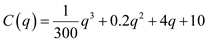(a) A perfectly competitive industry is in long-run equilibrium if there are no incentives for profit-maximizing firms to enter or to leave the industry. This will occur when (a) the number of firms is such that P = MC = AC and (b) each firm operates at the low point of its long-run average cost curve. The calculation of supply curve is as follows:The firm's short-run supply curve with q as a function of market price (P) is given as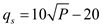. (b) Assume that there are no interaction effects among costs of the firms in the industry, the short-run industry supply curve is given as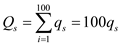Now here; then the industry supply curve is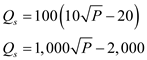Thus, the industry supply curve is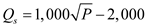. (c) Suppose market demand is given by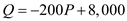In short run equilibrium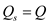, That isAssume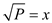and solve the quadratic equation. The calculation is shown below: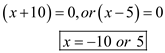Thus, the value of x is either -10 or 5. Ignore the negative value as price cannot be negative, thus the value of x is 5. Thus, the value of P is \$25 per unit (because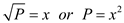) Substitute this value of price in either the demand function or the supply function. The calculation of equilibrium quantity is as follows:Therefore, the equilibrium price is \$25 per unit and the equilibrium quantity is 3,000 units.

Perfect Competition is a market structure where large numbers of sellers sell their goods and services to the buyer. Firm operating in this market are price takers as the price of good or service is decided by market forces and they are operating in zero profit situations. The market is highly competitive because entry and exit of firms are free in the market. As per the given information, there are 1000 firms producing diamonds in the market. The total cost function for each firm is given below: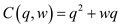Where,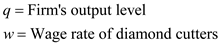a. It is given that w is 10. The value of w is 10 is put in the cost function. So, the function is now written as: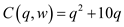It is required to calculate firm's short run supply curve and supply curve of industry. In order to calculate the supply curve of a firm, first Marginal Cost (MC) is computed as shown below:Let price of diamonds equal to P. Now, the firm's supply curve is calculated. Price of diamonds is equated to Marginal Cost as shown below:Therefore, firm's supply curve is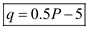Industry's supply is the summation of the individual supply of each seller. The industry's supply curve can be calculated as shown below: Let the industry output be denoted by Q.Thus, the industry supply curve is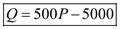. At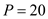, the diamonds produced are calculated below:The diamonds produced atare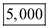. For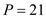, the diamonds produced are calculated below:The diamonds produced atare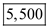. b. Now, wage is a function of output produced by industry. The function is given as: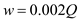Which is put in the cost function. So, the cost function is now written as: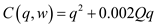It is required to calculate firm's short run supply and supply curve of industry. In order to calculate the supply curve of a firm, first Marginal Cost (MC) is computed as shown below:It is seen clearly that the firm's marginal cost is a function of Q. Let price of diamonds equal to P. Now, the firm's supply curve is calculated. Price of diamonds is equated to Marginal Cost as shown below:Therefore, firm's supply curve is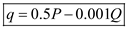Industry's supply is the summation of the individual supply of each seller. The industry's supply curve can be calculated as shown below: Let, the industry's output is denoted by Q.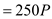Thus, the industry's supply curve is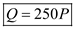. At, the diamonds produced are calculated below:The diamonds produced atare. At, the diamonds produced are calculated below:The diamonds produced atare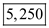. The short-run supply curve is steeper when wage rate is a function of total quantity demanded by the industry Q. With same change in price, changes quantity demanded to 250 units as compared to 500 units when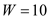. This is because on second case wage rate is a function of Q.

Perfect Competition is a market structure where large numbers of sellers sell their goods and services to buyer. Firm operates in this market are price takers as price of good or service is decided by market forces and they are operating in zero profit situations. The market is highly competitive because entry and exit of firms is free in the market. As per the question, there are 1,000 firms in a perfect competitive market. The market demand (Q) is given as: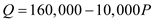And supply for each firm is given as 100 units. The total market supply (S) can be computed as shown below:Thus, market supply is 100,000 units. a. In order to calculate the equilibrium price, the market demand and market supply are equated. The equilibrium price can be calculated as shown below:Therefore, the equilibrium price is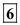. b. The market demand is given as:The demand for a single firm is calculated as shown below:Now, the demand schedule can be obtained by putting various values in the Individual Demand equation. The same is shown below: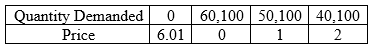c. When one seller in the market decides not to sell, the market supply reduces to 99,900 units. The calculation of the new equilibrium price is shown below:Hence, the new equilibrium price is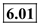. When one seller decides to sell 200 units, the market supply changes to 100,100 units. The calculation of the new equilibrium price is shown below:Hence, the new equilibrium price is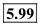. d. The elasticity of industry demand curve at equilibrium point can be computed as follows:Hence, the elasticity of industry demand curve is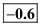. The elasticity for any one seller at equilibrium point can be computed as shown below:Hence, the elasticity of industry demand curve is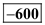. Now, the supply curve (q i ) for single firm is given as: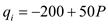The supply curve for market can be computed as shown below:Thus, market supply is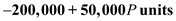. a. In order to calculate the equilibrium price, the market demand and market supply are equated. The equilibrium price can be calculated as shown below:Therefore, the equilibrium price is. b. The market demand is given as:The demand for a single firm is calculated as shown below:Now, the demand schedule can be obtained by putting various values in the Individual Demand equation. The same is shown below: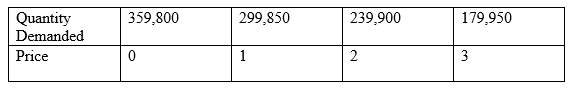c. When one seller in the market decides not to sell, the market supply reduces to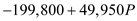units. The calculation of the new equilibrium price is shown below:Hence, the new equilibrium price is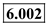. When one seller decides to sell 200 units, the market supply changes to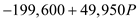units. The calculation of the new equilibrium price is shown below:Hence, the new equilibrium price is. d. The elasticity of industry demand curve at equilibrium point can be computed as follows:Hence, the elasticity of industry demand curve is. The elasticity for any one seller at equilibrium point can be computed as shown below:Hence, the elasticity of industry demand curve is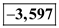.